## 5.7 One with proof by cases.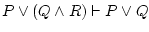It will be needed the most complex derivation rule: the disjunction elimination.solved: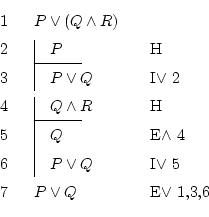You already know the rules, so I just explain the way of thinking of a human who doesn't know natural deduction but can think a little:

We need to know that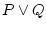is always true. The expression from the left,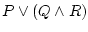, can be made true because of two causes:

• if it's true because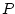is true, thenis true.
• if it's true because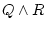is true, then both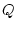and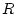are true, sois true because of.
So, anyway,is true.

Well, now we just need to translate all this to logical language, following the same order in which we thought that, and proceeding slowly.

We start proving one path, then the other, and finally we apply the disjunction elimination. To justify it we must write the line where the disjunction is in, and the two lines from inside each subdemonstration where we saw that both supposing one thing or supposing the other leads to the same result.

Notice that, even if we discovered that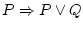and that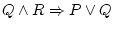, it isn't necessary to use implication introduction to keep this written down.

The hardest thing in proof by cases is to decide which will be the expression to prove in both cases. It must be exactly the same in both cases!

Daniel Clemente Laboreo 2005-05-17